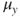# Chapter 4Univariate meta-analysis

This chapter begins by introducing the basic ideas of the fixed-effects model. The extension to the random-effects model is then introduced. Conceptual and statistical differences between the fixed-effects and the random-effects models are discussed. By including study characteristics as moderators, we extend the random-effects model to the mixed-effects model. Key concepts in a meta-analysis are introduced, such as testing the homogeneity of effect sizes, estimating heterogeneity variance, quantifying the degree of heterogeneity in the random-effects model, and quantifying the explained variance in the mixed-effects model. These models are then formulated under the structural equation modeling (SEM) framework. This SEM-based meta-analysis provides the foundation for more advanced analyses such as the multivariate and the three-level meta-analyses introduced in later chapters. Graphical models are proposed to represent the meta-analytic models. Several applications are used to illustrate the procedures in the R statistical environment.

## 4.1 Introduction

We begin this chapter by considering a model with only one variablethat is normally distributed with a mean ofand a variance of , that is, . As we are going to show later, this simple model has ...

Get Meta-Analysis: A Structural Equation Modeling Approach now with the O’Reilly learning platform.

O’Reilly members experience live online training, plus books, videos, and digital content from nearly 200 publishers.